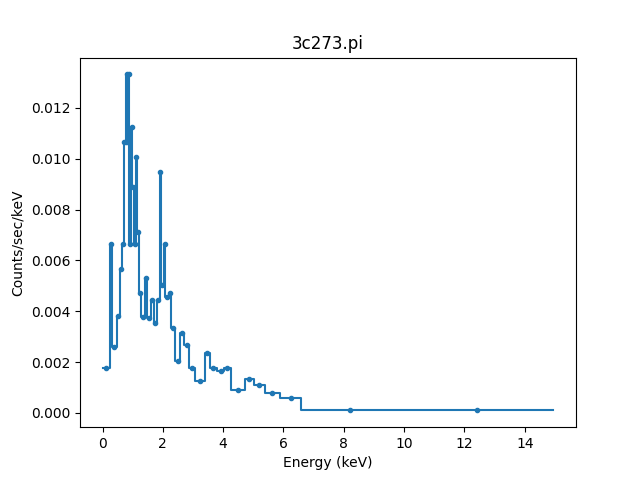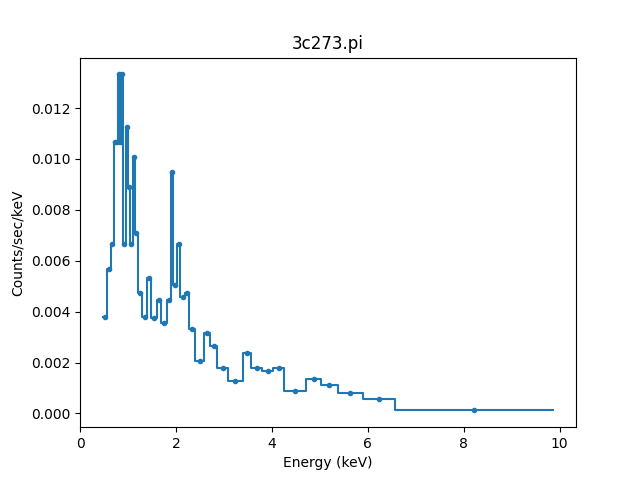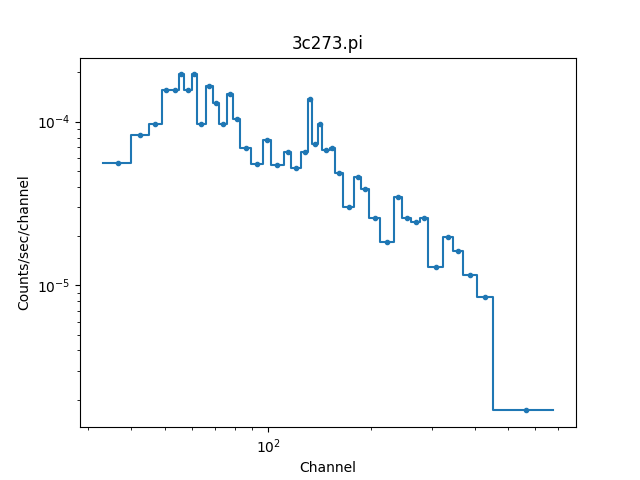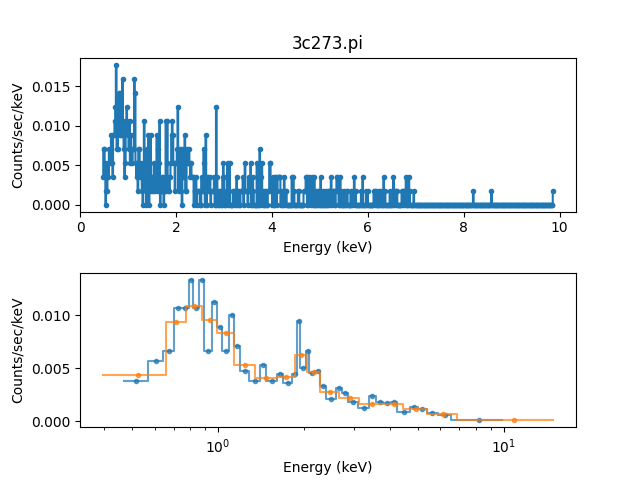# Analyzing PHA data

As mentioned in Accessing filtered data, PHA datasets add extra features to the standard Sherpa data class. These are:

• creating a PHA data set requires significantly more metadata than other data classes, which is automatically handled when reading the data from a FITS file that follows the OGIP standards, but have to be explicitly set when creating a `DataPHA` object;

• the `sherpa.astro.data.DataPHA` class is derived from `sherpa.data.Data1D`, since the “raw” data being fit is channel number and counts, but much analysis requires an “integrated” data set;

• a source region can also have one or more associated background regions which provide an estimate of the background contamination in the source region and this can either be subtracted from the data or a separate model fit to it;

• analysis can be done using one of three “coordinates” - `"channel"`, `"energy"`, or `"wavelength"` - that control the plot appearance and units for the `notice()` and `ignore()` calls;

• data can be dynamically regrouped to change the signal-to-noise during the analysis;

• and model evaluation uses classes related to the ARF and RMF to convert between channels and energies.

The X-ray data (DataPHA) section of the model evaluation page shows how many of these features work, while below we focus on an overview of these changes.

The following imports have been made:

```>>> import numpy as np
>>> from matplotlib import pyplot as  plt
>>> from sherpa.astro.data import DataPHA
```

## Creating a DataPHA object

The `sherpa.astro.io.read_pha()` routine will read in a PHA FITS file:

```>>> from sherpa.astro.io import read_pha
statistical errors were found in file '3c273.pi'
but not used; to use them, re-read with use_errors=True
statistical errors were found in file '3c273_bg.pi'
but not used; to use them, re-read with use_errors=True
>>> print(pha)
name           = 3c273.pi
channel        = Float64
counts         = Float64
staterror      = None
syserror       = None
bin_lo         = None
bin_hi         = None
grouping       = Int16
quality        = Int16
exposure       = 38564.608926889
backscal       = 2.5264364698914e-06
areascal       = 1.0
grouped        = True
subtracted     = False
units          = energy
rate           = True
plot_fac       = 0
response_ids   = 
background_ids = 
```

Note

The `sherpa.astro.io` module requires that a FITS backend is available. The Astropy package can be used for this.

As well as reading in the data it has also automatically loaded in the background data and response information (ARF and RMF) that are set in this file’s FITS metdata:

```>>> print(pha.get_background())
name           = 3c273_bg.pi
channel        = Float64
counts         = Float64
staterror      = None
syserror       = None
bin_lo         = None
bin_hi         = None
grouping       = Int16
quality        = Int16
exposure       = 38564.608926889
backscal       = 1.872535141462e-05
areascal       = 1.0
grouped        = True
subtracted     = False
units          = energy
rate           = True
plot_fac       = 0
response_ids   = 
background_ids = []
>>> print(pha.get_arf())
name     = 3c273.arf
energ_lo = Float64
energ_hi = Float64
specresp = Float64
bin_lo   = None
bin_hi   = None
exposure = 38564.141454905
ethresh  = 1e-10
>>> print(pha.get_rmf())
name     = 3c273.rmf
detchans = 1024
energ_lo = Float64
energ_hi = Float64
n_grp    = UInt64
f_chan   = UInt64
n_chan   = UInt64
matrix   = Float64
offset   = 1
e_min    = Float64
e_max    = Float64
ethresh  = 1e-10
```

A PHA object can also be created directly - all that is needed at first are the channel and counts arrays as other metadata can be added after the `DataPHA` object has been created:

```>>> chans = np.arange(1, 1025, dtype=int)
>>> counts = np.ones(1024, dtype=int)
>>> test = DataPHA('example', chans, counts)
>>> print(test)
name           = example
channel        = Int64
counts         = Int64
staterror      = None
syserror       = None
bin_lo         = None
bin_hi         = None
grouping       = None
quality        = None
exposure       = None
backscal       = None
areascal       = None
grouped        = False
subtracted     = False
units          = channel
rate           = True
plot_fac       = 0
response_ids   = []
background_ids = []
```

## Visualizing the data

The `sherpa.astro.plot` module contains classes for visualizing the data (the visualization section provides more information on how to use the plot classes), so we can visualize the data with:

```>>> from sherpa.astro.plot import DataPHAPlot
>>> plot = DataPHAPlot()
>>> plot.histo_prefs['linestyle'] = '-'
>>> plot.prepare(pha)
>>> plot.plot()
```Note

The `sherpa.astro.plot` module requires that a plotting backend is available. The matplotlib package can be used for this.

The default plot style has been adjusted to also include the bin edges (by setting the matplotlib `linestyle` option).

## Analysis units

The “native” units for analyzing PHA data is in channel space, but this is often not the most instructive, so normally the analysis is done with energy or wavelength units. If a PHA file is loaded in from disk and contains a response then it will default to energy units, otherwise it will use channel units.

For this example, the `3c273.pi` file includes response information in its header - via the `RESPFILE` and `ANCRFILE` keywords - and so the selected units are `energy`, which can be found using either the `get_analysis()` method or the `units` attribute:

```>>> pha.get_analysis()
'energy'
>>> print(pha.units)
energy
```

The `set_analysis` method or `units` attribute can be used to switch between `channel`, `energy`, and `wavelength` (the latter two will raise an error if no response has been set).

## Filtering

The default is for all the data to be included and, because the file contained grouping information, the data has been grouped (this is shown in the plot above, where there are only of order 50 data points shown rather than the 1024 channels this data set has):

```>>> print(pha.mask)
True
>>> print(pha.grouped)
True
```

The “dependent axis” - so in this case, the counts - can be retrieved with the `get_dep()` method, and we can see the difference the `filter` flag makes:

```>>> y1 = pha.get_dep()
>>> y2 = pha.get_dep(filter=True)
>>> print(y1.size)
1024
>>> print(y2.size)
46
```

So when `filter=False` (the default) then the ungrouped data is returned, but when `filter=True` the grouped data is returned.

Since the analysis units for this data set are `energy`, we can select a subset of the data, which means that the grouped data size is reduced, as the points with energies below 0.5 keV or above 7 keV have been removed:

```>>> pha.notice(0.5, 7)
>>> print(pha.get_dep(filter=True).size)
42
>>> plot.prepare(pha)
>>> plot.plot()
```Although the requested range was 0.5 to 7.0 keV, the selected range is wider, as shown above and with the `get_filter()` method:

```>>> print(pha.get_filter())
0.467200011015:9.869600296021
```

Note

Each channel covers a finite energy range, and so when determining what value to display, the `get_filter()` call uses the full range (this was changed in Sherpa 4.14.0, in earlier versions the mid-point was used so the expression would appear to cover a smaller range but would still reflect the same filter).

The reason for this change is two fold:

• as mentioned, each channel has a finite energy range, so the selected energy range is unlikely to exactly match the requested range,

• and thanks to grouping, the selected channel is unlikely to fall at the start (for the low limit) and end (for the high limit) values for the groups, so this further changes the selected limit range.

Consider the following highly-simplified case where there are 7 channels that have been grouped into 4 bins.

Channel

Group

Energy range (keV)

1

1

0.10 - 0.11

2

0.11 - 0.14

3

2

0.14 - 0.16

4

0.16 - 0.20

5

3

0.20 - 0.22

6

0.22 - 0.24

7

4

0.24 - 0.26

In this case a filter to notice the range 0.15 to 0.21 keV would select channels 3 to 5 and then end up selecting groups 2 and 3, with the final channel selection being 3 to 6. A call to then ignore the range 0.18 to 0.19 keV would select channel 4 and hence group 2, so resulting in a final filter of just group 3 (channels 5 to 6).

We can switch temporarily to channel units and see differences in the `get_filter` call and the plot:

```>>> pha.units = 'channel'
>>> print(pha.get_filter())
33:676
>>> plot.prepare(pha)
>>> plot.plot(xlog=True, ylog=True)
>>> pha.units = 'energy'
```Note

The counts (dependent) axis is drawn with a logarithmic scale primarily because the values are small enough that the Y-axis label would disappear with a linear scale. The channel (independent) axis has been drawn with a log scale because the effective area of this instrument is higher at lower energies which tends to result in smaller groups at low channel values.

### Filtering and limits

When using energy or wavelength units - e.g. with `set_analysis()`, as described above - the meaning of the arguments to `notice()` and `ignore()` are slightly different than when using channel units. When using channels:

• the low and high limits must be integers,

• and the limits are inclusive on both ends (so `[lo, hi]`)

whereas for energy and wavelength analysis

• the low and high limits must be >= 0,

• and the limits are only inclusive on the lower value (so `[lo, hi)`, that is a half-open interval).

That is (for an ungrouped dataset):

```>>> pha.notice()
>>> pha.units = 'channel'
>>> pha.notice(20, 200)
```

will select the channels 20 to 200 (inclusive), but

```>>> pha.notice()
>>> pha.units = 'energy'
>>> pha.notice(0.5, 7.0)
```

will only select those bins which lie in the range ```0.5 <= energy < 7.0``` (although as just explained, the finite width of each channel in energy or wavelength units means that the distinction between `0.5 <= energy < 7.0` and ```0.5 <= energy <= 7.0``` makes no difference unless the bin edge is at 7 keV).

Note

The PHA filtering in Sherpa 4.14.0 has been updated to fix a number of corner cases which can result in filter expressions changing the first or last selected bin. This can then cause fit differences as the number of degrees of freedom and the fit parameters can change slightly.

## Grouping

The dynamic grouping can be changed by setting the `grouping` attribute - and then calling the `group()` if necessary - or with one of the dynamic-routines methods: `group_adapt()`, `group_adapt_snr()`, `group_bins()`, `group_counts()`, `group_snr()`, and `group_width()`.

For this example we will compare the same data with different grouping schemes by loading the data in three times. The `SherpaVerbosity` class is used to temporarily hide the output of `read_pha()`:

```>>> from sherpa.utils.logging import SherpaVerbosity
>>> with SherpaVerbosity('ERROR'):
```

The same energy range will be used for all three data sets:

```>>> pha1.notice(0.5, 7)
>>> pha2.notice(0.5, 7)
>>> pha3.notice(0.5, 7)
```

The first data set is to be ungrouped, the second data set will use the on-disk grouping settings, and the third data set is grouped so that each bin contains at least 40 counts:

```>>> pha1.ungroup()
>>> pha3.group_counts(40)
```

For display the ungrouped data is shown in a separate plot as it makes it easier to compare:

```>>> plt.subplot(2, 1, 1)
>>> plot.prepare(pha1)
>>> plot.plot(clearwindow=False)
>>> plt.subplot(2, 1, 2)
>>> plot.prepare(pha2)
>>> plot.plot(xlog=True, alpha=0.7, clearwindow=False)
>>> plot.prepare(pha3)
>>> plot.overplot(alpha=0.7)
>>> plt.title('')
```In general, as the grouped bins become larger then the difference of the filtered range to the requested range becomes larger:

```>>> print(pha1.get_filter())
0.467200011015:9.869600296021
>>> print(pha2.get_filter())
0.467200011015:9.869600296021
>>> print(pha3.get_filter())
0.394199997187:14.950400352478
```

## Manipulating data

Methods like `get_dep()` will apply the necessary grouping and filters to the data. It can be useful to convert other arrays - such as counts or energy bins - directly, which can be done with `apply_filter()` and `apply_grouping()`. The default behavior for `apply_filter` is to sum the data values with each group, so we can re-create the `get_dep` call:

```>>> d1 = pha.get_dep(filter=True)
>>> d2 = pha.apply_filter(pha.counts)
>>> np.all(d1 == d2)
True
```

The behavior can be changed with the `groupfunc` argument, which takes a limited set of functions that describe how the data within a group is combined (the default is `np.sum`). For instance, the first and last channel value of each group can be calculated with:

```>>> clo = pha.apply_filter(pha.channel, groupfunc=pha._min)
>>> chi = pha.apply_filter(pha.channel, groupfunc=pha._max)
>>> clo[0:7]
[33. 40. 45. 49. 52. 55. 57.]
>>> chi[0:7]
[39. 44. 48. 51. 54. 56. 59.]
```

The `apply_grouping()` method is similar but it does not apply any filter, so all channels are used. So to get the group boundaries for all channels, not just the filtered ones, we can say:

```>>> alo = pha.apply_grouping(pha.channel, pha._min)
>>> ahi = pha.apply_grouping(pha.channel, pha._max)
>>> alo[0:7]
[ 1. 18. 22. 33. 40. 45. 49.]
>>> ahi[0:7]
[17. 21. 32. 39. 44. 48. 51.]
```

## Background

A PHA data set may have one or more associated background data sets. For this example there is one, and the `get_background()` method will return a `DataPHA` object representing the background region.

```>>> print(pha.background_ids)

>>> bkg = pha.get_background()
>>> print(bkg)
name           = 3c273_bg.pi
channel        = Float64
counts         = Float64
staterror      = None
syserror       = None
bin_lo         = None
bin_hi         = None
grouping       = Int16
quality        = Int16
exposure       = 38564.608926889
backscal       = 1.872535141462e-05
areascal       = 1.0
grouped        = True
subtracted     = False
units          = energy
rate           = True
plot_fac       = 0
response_ids   = 
background_ids = []
```

Note

In this example the background data has the same exposure time as the source, which is often the case (the source and background spectra are extracted from the same event file), but this does not need to hold.

Often all that is done is to subtract the background from the source data, which is achieved with the `subtract()` method, but you can instead fit a model to just the background data, and have this then included in the source region (with appropriate scaling to account for differences in the source and background apertures). Filtering and grouping changes to a source region are automatically propogated to the associated background regions, but they can be applied to the background data set directly if needed.

For example, the un-subtracted group counts are:

```>>> pha.get_dep(filter=True)
[15. 16. 15. 18. 18. 15. 18. 15. 15. 19. 15. 15. 17. 16. 16. 17. 15. 19.
15. 16. 15. 16. 17. 15. 18. 16. 15. 15. 16. 15. 15. 15. 16. 16. 15. 15.
16. 16. 15. 16. 15. 15.]
```

and after background subtractuion the values are:

```>>> pha.subtract()
>>> pha.get_dep(filter=True)
[14.86507936 15.86507936 15.         18.         18.         14.86507936
17.86507936 15.         14.59523807 18.86507936 14.86507936 14.86507936
17.         16.         15.73015871 16.73015871 15.         18.59523807
14.86507936 15.59523807 14.86507936 16.         16.59523807 15.
17.59523807 16.         15.         14.46031742 16.         14.59523807
14.59523807 14.46031742 15.86507936 15.59523807 14.32539678 14.32539678
15.32539678 15.19047614 14.32539678 15.19047614 14.05555549 12.43650777]
```